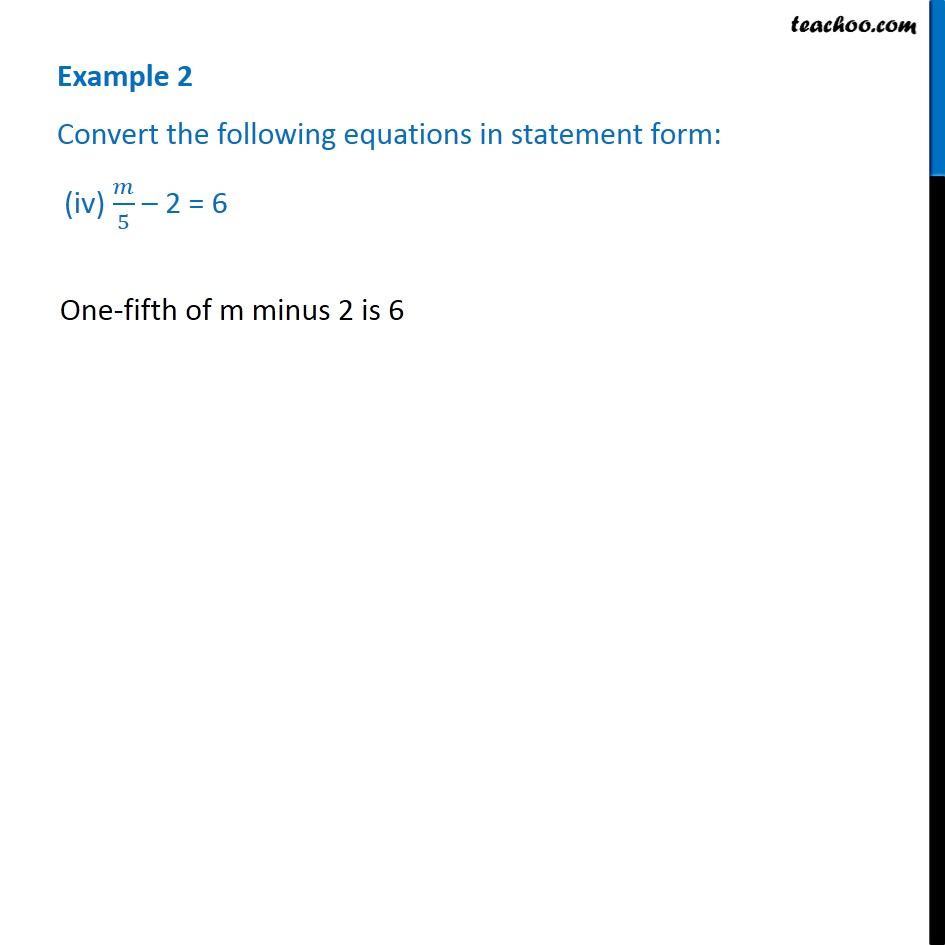Examples

Chapter 4 Class 7 Simple Equations
Serial order wiseGet live Maths 1-on-1 Classs - Class 6 to 12

### Transcript

Example 2 Convert the following equations in statement form: (iv) 𝑚/5 – 2 = 6 One-fifth of m minus 2 is 6